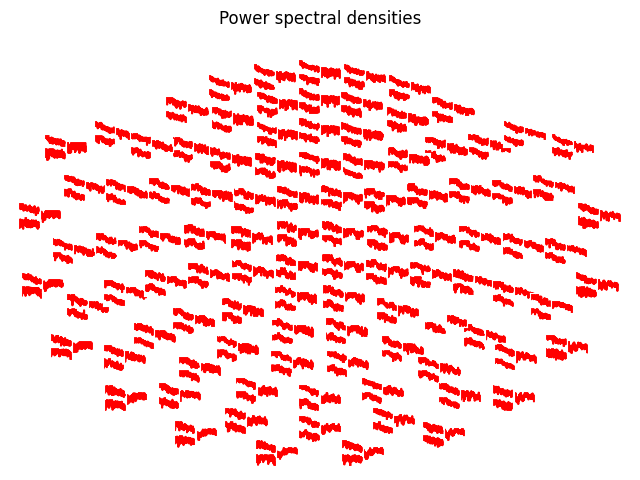# Plot custom topographies for MEG sensors#

This example exposes the `iter_topography()` function that makes it very easy to generate custom sensor topography plots. Here we will plot the power spectrum of each channel on a topographic layout.

```# Author: Denis A. Engemann <denis.engemann@gmail.com>
#
```
```import numpy as np
import matplotlib.pyplot as plt

import mne
from mne.viz import iter_topography
from mne import io
from mne.datasets import sample

print(__doc__)

data_path = sample.data_path()
meg_path = data_path / "MEG" / "sample"
raw_fname = meg_path / "sample_audvis_filt-0-40_raw.fif"

raw.filter(1, 20, fir_design="firwin")

picks = mne.pick_types(raw.info, meg=True, exclude=[])
tmin, tmax = 0, 120  # use the first 120s of data
fmin, fmax = 2, 20  # look at frequencies between 2 and 20Hz
n_fft = 2048  # the FFT size (n_fft). Ideally a power of 2
spectrum = raw.compute_psd(picks=picks, tmin=tmin, tmax=tmax, fmin=fmin, fmax=fmax)
psds, freqs = spectrum.get_data(exclude=(), return_freqs=True)
psds = 20 * np.log10(psds)  # scale to dB

def my_callback(ax, ch_idx):
"""Handle axes callback.

This block of code is executed once you click on one of the channel axes
in the plot. To work with the viz internals, this function should only take
two parameters, the axis and the channel or data index.
"""
ax.plot(freqs, psds[ch_idx], color="red")
ax.set_xlabel("Frequency (Hz)")
ax.set_ylabel("Power (dB)")

for ax, idx in iter_topography(
raw.info,
fig_facecolor="white",
axis_facecolor="white",
axis_spinecolor="white",
on_pick=my_callback,
):
ax.plot(psds[idx], color="red")

plt.gcf().suptitle("Power spectral densities")
plt.show()
``````Opening raw data file /home/circleci/mne_data/MNE-sample-data/MEG/sample/sample_audvis_filt-0-40_raw.fif...
Read a total of 4 projection items:
PCA-v1 (1 x 102)  idle
PCA-v2 (1 x 102)  idle
PCA-v3 (1 x 102)  idle
Average EEG reference (1 x 60)  idle
Range : 6450 ... 48149 =     42.956 ...   320.665 secs
Reading 0 ... 41699  =      0.000 ...   277.709 secs...
Filtering raw data in 1 contiguous segment
Setting up band-pass filter from 1 - 20 Hz

FIR filter parameters
---------------------
Designing a one-pass, zero-phase, non-causal bandpass filter:
- Windowed time-domain design (firwin) method
- Hamming window with 0.0194 passband ripple and 53 dB stopband attenuation
- Lower passband edge: 1.00
- Lower transition bandwidth: 1.00 Hz (-6 dB cutoff frequency: 0.50 Hz)
- Upper passband edge: 20.00 Hz
- Upper transition bandwidth: 5.00 Hz (-6 dB cutoff frequency: 22.50 Hz)
- Filter length: 497 samples (3.310 s)

[Parallel(n_jobs=1)]: Done  17 tasks      | elapsed:    0.0s
[Parallel(n_jobs=1)]: Done  71 tasks      | elapsed:    0.1s
[Parallel(n_jobs=1)]: Done 161 tasks      | elapsed:    0.3s
[Parallel(n_jobs=1)]: Done 287 tasks      | elapsed:    0.5s
Effective window size : 13.639 (s)
```

Total running time of the script: (0 minutes 11.655 seconds)

Estimated memory usage: 164 MB

Gallery generated by Sphinx-Gallery